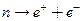Chapter 33, Problem 28PE

Chapter
Section
Textbook Problem

Is the decaypossible considering the appropriate conservation laws? State why or why not.

To determine

Whether the decay ne++e is possible as per the conservation laws.

Explanation

Given info:

Given reaction is ne++e

Calculation:

We can verify whether the decay ne++e is possible by checking the following conservation laws.

Decay:ne++e

Charge: 0  +11conserved

B:  +1

Still sussing out bartleby?

Check out a sample textbook solution.

See a sample solution

The Solution to Your Study Problems

Bartleby provides explanations to thousands of textbook problems written by our experts, many with advanced degrees!

Get Started